Related Articles
• Explore Our Geeks Community
• Data visualization with R and ggplot2

# Modify axis, legend, and plot labels using ggplot2 in R

In this article, we are going to see how to modify the axis labels, legend, and plot labels using ggplot2 bar plot in R programming language.

For creating a simple bar plot we will use the function geom_bar( ).

Syntax: geom_bar(stat, fill, color, width)

Parameters :
stat : Set the stat parameter to identify the mode.
fill : Represents color inside the bars.
color : Represents color of outlines of the bars.
width : Represents width of the bars.

Dataset in use: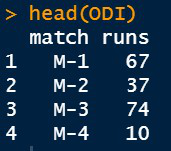Let us first visualize the graph as it is so that the changes are apparent.

Example:

## R

 `# Inserting data` `ODI <- ``data.frame``(match=``c``(``"M-1"``,``"M-2"``,``"M-3"``,``"M-4"``),` `                   ``runs=``c``(67,37,74,10))` `head``(ODI)`   `library``(ggplot2)`   `# Default axis labels in ggplot2 bar plot` `perf <-``ggplot``(data=ODI, ``aes``(x=match, y=runs,fill=match))+` `  ``geom_bar``(stat=``"identity"``)`   `perf`

Output:### Adding axis labels and main title in the plot

By default, R will use the variables provided in the Data Frame as the labels of the axis. We can modify them and change their appearance easily. The functions which are used to change axis labels are :

• xlab( ) : For the horizontal axis.
• ylab( ) : For the vertical axis.
• labs( ) : For both the axes simultaneously.
• element_text( ) : The arguments of this function are :

Syntax:

element_text( family, face, color, size, hjust, vjust, angle, margin)

• element_blank( ): To make the labels NULL and remove them from the plot.

The argument hjust (Horizontal Adjust) or vjust (Vertical Adjust) is used to move the axis labels. They take numbers in range [0,1] where :

hjust = 0 // Depicts left most corner of the axis

hjust = 0.5 // Depicts middle of the axis

hjust = 1 // Depicts right most corner of the axis

The keywords used are :

• title : To add plot label.
• subtitle : To add subtitle in the plot.
• caption :  To add caption in the plot.
• axis.title.x : For horizontal axis.
• axis.title.y : For vertical axis.

Example:

## R

 `library``(ggplot2)`   `# Inserting data` `ODI <- ``data.frame``(match=``c``(``"M-1"``,``"M-2"``,``"M-3"``,``"M-4"``),` `                   ``runs=``c``(67,37,74,10))`   `# Default axis labels in ggplot2 ` `# bar plot` `perf <-``ggplot``(data=ODI, ``aes``(x=match, y=runs,fill=match))+` `  ``geom_bar``(stat=``"identity"``)` `perf`   `# Manually adding axis labels and ` `# Plot Label` `ggp<-perf+``labs``(x=``"Matches"``,y=``"Runs Scored"``,` `               ``title=``"Runs scored by Virat Kohli in ODI matches"``)` `ggp`

Output: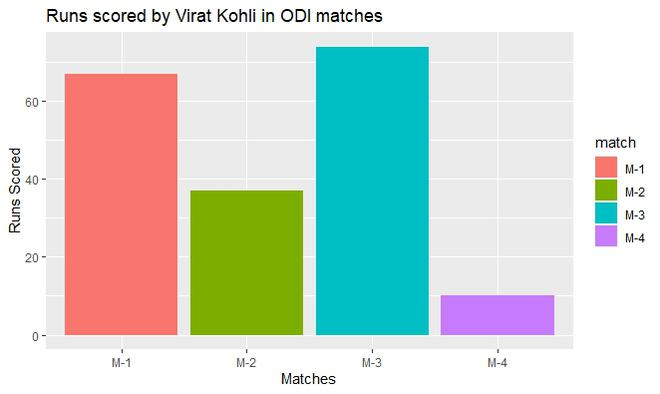A subtitle can also be included with the main title using labs() function and passing subtitle argument with the required subtitle.

Example:

## R

 `library``(ggplot2)`   `# Inserting data` `ODI <- ``data.frame``(match=``c``(``"M-1"``,``"M-2"``,``"M-3"``,``"M-4"``),` `                   ``runs=``c``(67,37,74,10))`   `# Default axis labels in ggplot2 bar plot` `perf <-``ggplot``(data=ODI, ``aes``(x=match, y=runs,fill=match))+` `  ``geom_bar``(stat=``"identity"``)` `perf`   `# Manually adding axis labels and Plot Label` `ggp<-perf+``labs``(x=``"Matches"``,y=``"Runs Scored"``,` `               ``title=``"Runs scored by Virat Kohli in ODI matches"``)` `ggp`   `# Subtitle and Caption` `ggp+``labs``(subtitle=``"Performance"``,caption=``"GeeksforGeeks Trophy"``)`

Output: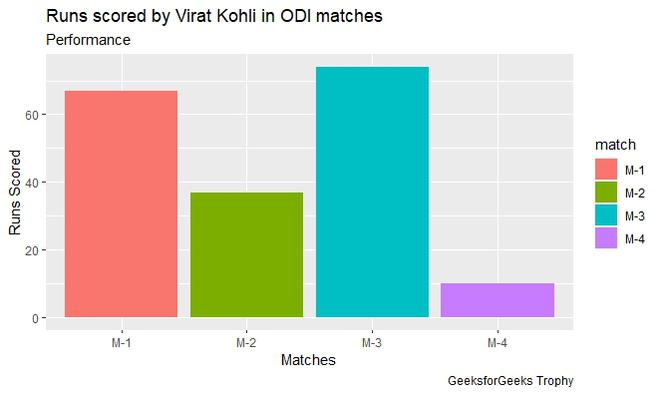To move axis labels hjust argument is set according to the requirement.

Example:

## R

 `library``(ggplot2)`   `# Inserting data` `ODI <- ``data.frame``(match=``c``(``"M-1"``,``"M-2"``,``"M-3"``,``"M-4"``),` `                   ``runs=``c``(67,37,74,10))`   `# Default axis labels in ggplot2 bar plot` `perf <-``ggplot``(data=ODI, ``aes``(x=match, y=runs,fill=match))+` `  ``geom_bar``(stat=``"identity"``)` `perf`   `# Manually adding axis labels and Plot Label` `ggp<-perf+``labs``(x=``"Matches"``,y=``"Runs Scored"``,` `               ``title=``"Runs scored by Virat Kohli in ODI matches"``)` `ggp`   `# Moving axis label to left` `ggp + ``theme``(` `  ``axis.title.x = ``element_text``(hjust=0),` `  ``axis.title.y = ``element_text``(hjust=0)` `  ``)`   `# Moving axis label in middle` `ggp + ``theme``(` `  ``axis.title.x = ``element_text``(hjust=0.5),` `  ``axis.title.y = ``element_text``(hjust=0.5)` `)`   `# Moving axis label to right` `ggp + ``theme``(` `  ``axis.title.x = ``element_text``(hjust=1),` `  ``axis.title.y = ``element_text``(hjust=1)` `)`

Output: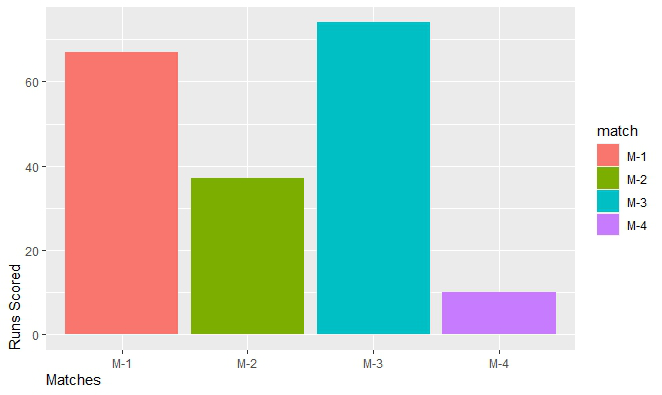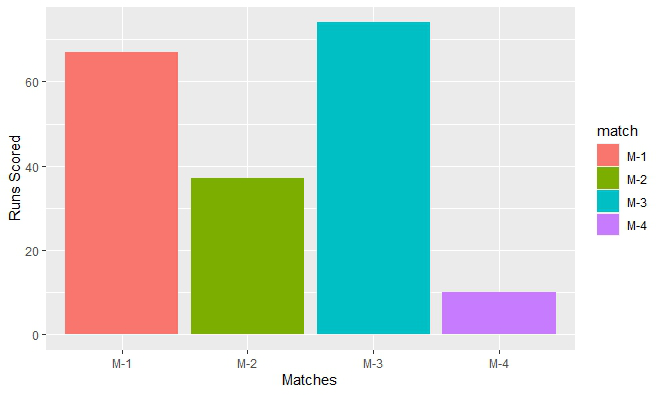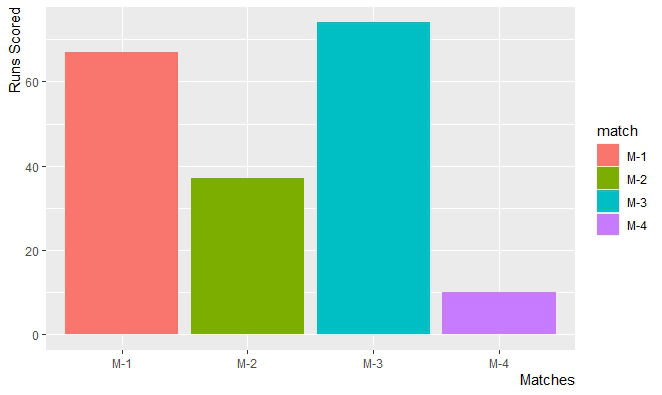### Formatting appearance of axis labels and main title of the plot

Axis labels and main titles can be changed to reflect the desired appearance. For this element_text() function is passed with the required attributes.

Example:

## R

 `library``(ggplot2)`   `# Inserting data` `ODI <- ``data.frame``(match=``c``(``"M-1"``,``"M-2"``,``"M-3"``,``"M-4"``),` `                   ``runs=``c``(67,37,74,10))`   `# Default axis labels in ggplot2 bar plot` `perf <-``ggplot``(data=ODI, ``aes``(x=match, y=runs,fill=match))+` `  ``geom_bar``(stat=``"identity"``)` `perf`   `# Manually adding axis labels and Plot Label` `ggp<-perf+``labs``(x=``"Matches"``,y=``"Runs Scored"``,` `               ``title=``"Runs scored by Virat Kohli in ODI matches"``)`   `# Plot title, axis labels format` `ggp + ``theme``(plot.title = ``element_text``(` `  ``colour=``"#006000"``, size=14,face=``"bold"``),` `            `  `            ``axis.title.x = ``element_text``(` `              ``colour=``"Purple"``,size=10,face=``"bold.italic"``),` `            `  `            ``axis.title.y = ``element_text``(` `              ``colour=``"DarkBlue"``,size=10,face=``"bold.italic"``)` `           ``)`

Output: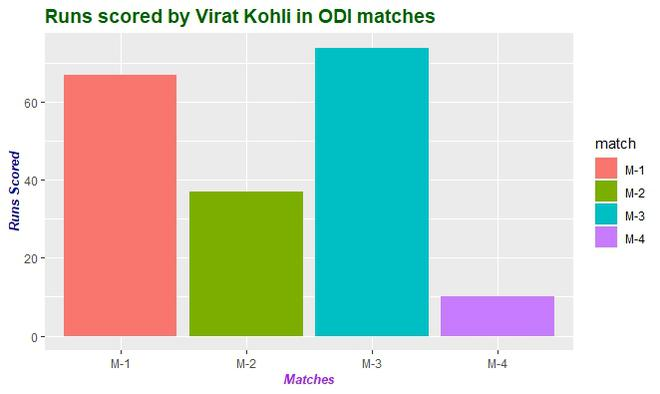### Removing the axis labels and plot the title

For this theme() function is called with reference to which part of the plot has to be modified. To these references, pass element_blank() without any argument.

Example:

## R

 `library``(ggplot2)`   `# Inserting data` `ODI <- ``data.frame``(match=``c``(``"M-1"``,``"M-2"``,``"M-3"``,``"M-4"``),` `                   ``runs=``c``(67,37,74,10))`   `# Default axis labels in ggplot2 bar plot` `perf <-``ggplot``(data=ODI, ``aes``(x=match, y=runs,fill=match))+` `  ``geom_bar``(stat=``"identity"``)` `perf`   `# Manually adding axis labels and Plot Label` `ggp<-perf+``labs``(x=``"Matches"``,y=``"Runs Scored"``,` `               ``title=``"Runs scored by Virat Kohli in ODI matches"``)`   `# Remove chart title and axis label` `ggp + ``theme``(plot.title = ``element_blank``(),` `            ``axis.title.x = ``element_blank``(),` `            ``axis.title.y = ``element_blank``()` `            ``)`

Output: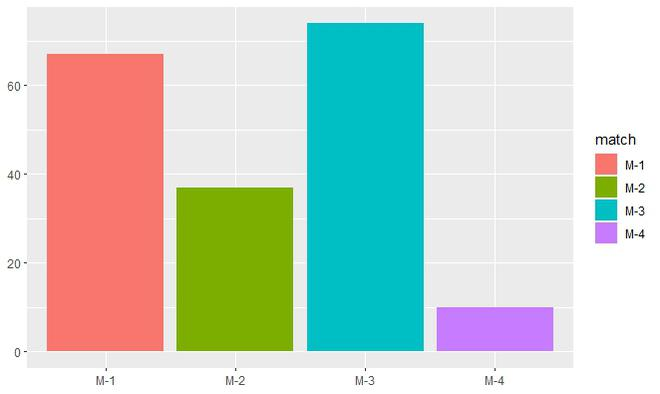### Changing the Position of the legends

To change the position of the legend theme() function is called with legend.position as argument and to this argument, a required position is passed.

Syntax:

theme( legend.position = “Pos”)

Parameter:

Pos : Left, Right, Top, Bottom.

Example:

## R

 `library``(ggplot2)`   `# Inserting data` `ODI <- ``data.frame``(match=``c``(``"M-1"``,``"M-2"``,``"M-3"``,``"M-4"``),` `                   ``runs=``c``(67,37,74,10))`   `# Default axis labels in ggplot2 bar plot` `perf <-``ggplot``(data=ODI, ``aes``(x=match, y=runs,fill=match))+` `  ``geom_bar``(stat=``"identity"``)` `perf`   `# Manually adding axis labels and Plot Label` `ggp<-perf+``labs``(x=``"Matches"``,y=``"Runs Scored"``,` `               ``title=``"Runs scored by Virat Kohli in ODI matches"``)`   `# Move legend to top position of the plot` `ggp + ``theme``(legend.position=``"top"``)`

Output: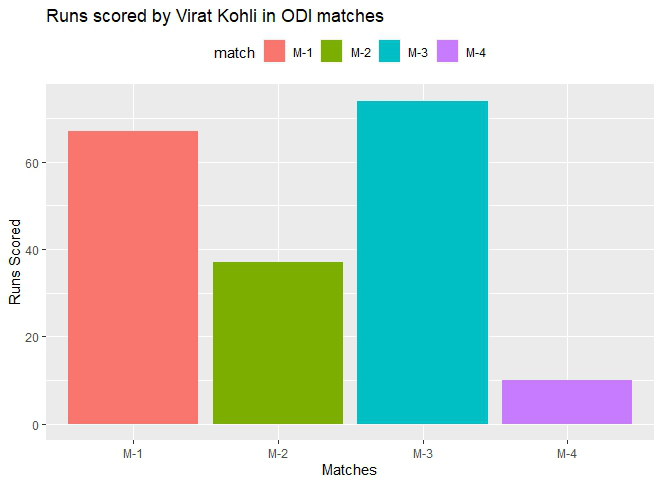Example:

## R

 `library``(ggplot2)`   `# Inserting data` `ODI <- ``data.frame``(match=``c``(``"M-1"``,``"M-2"``,``"M-3"``,``"M-4"``),` `                   ``runs=``c``(67,37,74,10))`   `# Default axis labels in ggplot2 bar plot` `perf <-``ggplot``(data=ODI, ``aes``(x=match, y=runs,fill=match))+` `  ``geom_bar``(stat=``"identity"``)` `perf`   `# Manually adding axis labels and Plot Label` `ggp<-perf+``labs``(x=``"Matches"``,y=``"Runs Scored"``,` `               ``title=``"Runs scored by Virat Kohli in ODI matches"``)`   `# Move legend to bottom position of the plot` `ggp + ``theme``(legend.position=``"bottom"``)`

Output: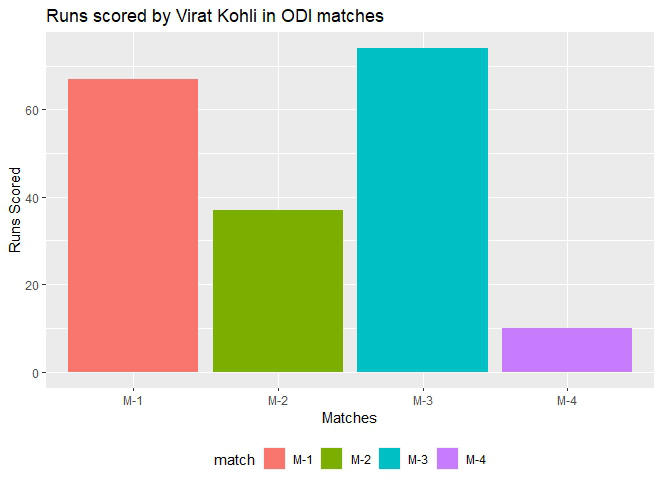Whether you're preparing for your first job interview or aiming to upskill in this ever-evolving tech landscape, GeeksforGeeks Courses are your key to success. We provide top-quality content at affordable prices, all geared towards accelerating your growth in a time-bound manner. Join the millions we've already empowered, and we're here to do the same for you. Don't miss out - check it out now!

Previous
Next